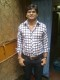# Array in C

Welcome to your Array in C

1. One-dimensional array needs only ____ subscript.
2. In ____ , address of the array elements are passed to function
3. In int x; the number 10 specified in the square bracket represents the
4. Fast access of array elements can be done using____
5. What is the output of int num={1,2,3,4}, {3,4,5,6} }; ?
6. The 1st element according to C compiler physically is a _____
7. In int x, the x represents a _____
8. When in between two arrays of the same data type, if the values are interchanged then _____
9. How many rows and columns are there in int num={ {1,2,3}, {4,5,6} }; ?
10. If in char c[]="Hello" the H is stored at 65470, then the o is stored at ___
11. Output of the following program isvoid main()
{
int x[]={1,2.2,3},i;
clrscr();
printf("%f",x);
}
12. In int x={2,3,4,5,6}; the base address is 65564, then the location of element 2 is ______
13. What is the value of a in static int  ; ?
14. An array is a collection of multiple elements of ____ data type
15. In int x if x=12 and x=26, then x is ____
16. Each array element is stored in separate
17. The array int x; can hold values in between_______ to ______
18. In long k the total memory occupied by the array is____
19. An array stores elements ____ in the memory
20. Array element counting starts from zero, hence the statement int x holds _____

## 2 thoughts on “Array in C”

1.Harsh pratap says:

It’s good to answer these questions.

1.response2vikash says:

Good,Thanks.